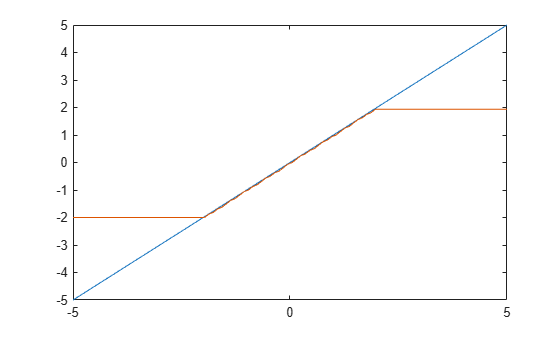# quantizenumeric

Quantize numeric data

## Syntax

``y = quantizenumeric(x,s,w,f)``
``y = quantizenumeric(x,s,w,f,r)``
``y = quantizenumeric(x,s,w,f,r,o)``

## Description

example

````y = quantizenumeric(x,s,w,f)` quantizes the value specified in `x` using signedness `s`, word length `w`, and fraction length `f`. Use `quantizenumeric` when you want to simulate full-precision arithmetic with doubles and then add quantization at certain steps in your algorithm without casting to fixed-point types.```

example

````y = quantizenumeric(x,s,w,f,r)` also specifies rounding mode `r`. ```

example

````y = quantizenumeric(x,s,w,f,r,o)` also specifies overflow mode `o`. ```

## Examples

collapse all

Quantize the value of pi using a signed numeric type with a word length of 16 bits, a fraction length of 13 bits, and rounding towards positive infinity.

```x = pi; y = quantizenumeric(x,1,16,13,'ceil')```
```y = 3.1416 ```

Specify a different rounding method. Observe that rounding towards zero affects the quantized value.

```x = pi; y = quantizenumeric(x,1,16,13,'fix')```
```y = 3.1415 ```

This example shows the effect of overflow action on the quantization of numeric data.

Create some data and quantize it with saturation on overflow specified.

```x = linspace(-5,5,100); y = quantizenumeric(x,1,6,4,'floor','saturate'); plot(x,x,x,y)```Change the overflow action to wrap on overflow and observe how the quantized data changes.

```z = quantizenumeric(x,1,6,4,'floor','wrap'); plot(x,x,x,z);```## Input Arguments

collapse all

Value to quantize, specified as a scalar, vector, matrix, or multidimensional array.

Data Types: `double`
Complex Number Support: Yes

Signedness of quantized value, specified as either `0` or `'false'` (unsigned) or `1` or `'true'` (signed).

Data Types: `double`

Word length of quantized value, specified as a positive scalar integer.

Data Types: `double`

Fraction length of quantized value, specified as a scalar integer.

Data Types: `double`

Rounding method to use for quantization, specified as a character vector:

• `'ceil'` — Round towards positive infinity (same as `'ceiling'`)

• `'ceiling'` — Round towards positive infinity (same as `'ceil'`)

• `'convergent'`— Convergent rounding

• `'fix'`— Round towards zero (same as `'zero'`)

• `'floor'`— Round towards negative infinity

• `'nearest'`— Round towards nearest with ties rounding towards positive infinity

• `'round'`— Round towards nearest with ties rounding up in absolute value

• `'zero'`— Round towards zero (same as `'fix'`)

Data Types: `char`

Overflow action to use for quantization, specified as either `'saturate'` or `'wrap'`.

Data Types: `char`

## Output Arguments

collapse all

Quantized output value, returned as a scalar, vector, matrix, or multidimensional array. `y` always has the same dimensions as `x` and is always a double.

## Version History

Introduced in R2016a

expand all

Behavior changed in R2021b